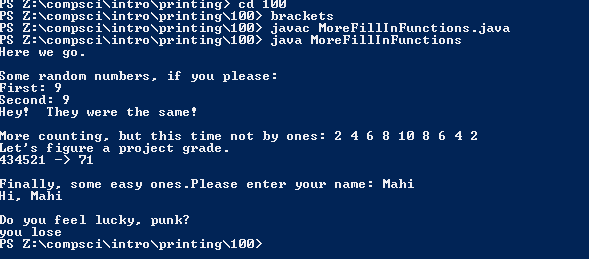# Assignment #100 and More Fill-In Functions

## Code

```    /// Name: Mahima Choudhary
/// Period: 6
/// Program Name: More Fill-In Functions
/// File Name: MoreFillInFunctions.java
/// Date Finished: 3/29/2016

// More Fill-In Functions - Fix the broken functions and function calls (again).

import java.util.Scanner;

public class MoreFillInFunctions
{
public static void main( String[] args )
{
// Fill in the function calls where indicated.
System.out.println("Here we go.");

System.out.println();
System.out.println("Some random numbers, if you please: ");
int lo, hi;
lo = 1;
hi = 10;
int val1 = superRand( lo, hi );
int val2 = superRand( hi, lo );

System.out.println("First: " + val1 );
// this time, put hi first
System.out.println("Second: " + val2 );
if ( val1 == val2 )
System.out.println("Hey!  They were the same!");
else
System.out.println("They were not the same.");

System.out.println();
System.out.print("More counting, but this time not by ones: ");
// count from 2 to 8 by 2s
stepCount( 2, 8, 2);
// count from 10 to 2 by 2s
stepCount( 10, 2, 2 );

System.out.println();
int a=4,b=3,c=4,d=5,e=2,f=1, result = projectGrade( a, b, c, d, e, f );
System.out.println("434521 -> " + result );

System.out.println();
System.out.print("Finally, some easy ones.");

String nombre = getName();
System.out.println("Hi, " + nombre );

System.out.println();
System.out.println("Do you feel lucky, punk?");
crash();
System.out.println();
}

public static int superRand( int a, int b )
// Parameters are:
//	int a;
//	int b;
{
// picks a number between a and b, no matter which is larger
int c;

if ( a < b )	// b is larger
c = a + (int)(Math.random()*(b-a+1));
else if ( a > b )	// a is larger
c = b + (int)(Math.random()*(a-b+1));
else
c = a;	// or c = b; doesn't matter since they're equal

return c;
}

public static int stepCount( int first, int last, int step )
// Parameters are:
//     int first;
//     int last;
//     int step;
{
// counts from 'first' to 'last' by 'step'
// handles forward and backward
int x;

if ( first < last )
{
x = first;
while ( x <= last )
{
System.out.print(x + " ");
x = x + step;
}
}
else
{
x = first;
while ( x >= last )
{
System.out.print(x + " ");
x = x - step;
}
}

return x;
}

public static int projectGrade( int p1, int p2, int p3, int p4, int p5, int p6 )
// Parameters are:
//     int p1, p2, p3, p4, p5, p6;
{
// given six integers representing scores out of five in each of the
// six categories for the first six weeks project: tells you your
// overall project grade out of 100

overall_grade = p1 * 6;    // six points per point for the first category
overall_grade = overall_grade + ( p2 * 6 );   // six points per point
overall_grade = overall_grade + ( p3 * 4 );   // four points per point
overall_grade = overall_grade + ( p4 * 2 );   // two points per point
overall_grade = overall_grade + ( p5 * 1 );   // one point per point
overall_grade = overall_grade + ( p6 * 1 );   // one point per point

}

public static String getName()
// No parameters.
{
// finds out the user's name
Scanner keyboard = new Scanner(System.in);

String name;
name = keyboard.next();

return name;
}

public static void crash()
// No parameters.
{
// displays "you win" or "you lose".  You lose 90% of the time.
String magic_word;

if ( (int)(Math.random()*10) == 0 )
{
// What do you know?  We won!
magic_word = "win";
}
else
{
// No big suprise; we lost.
magic_word = "lose";
}

System.out.print("you " + magic_word);
}
}
```

### Picture of the output